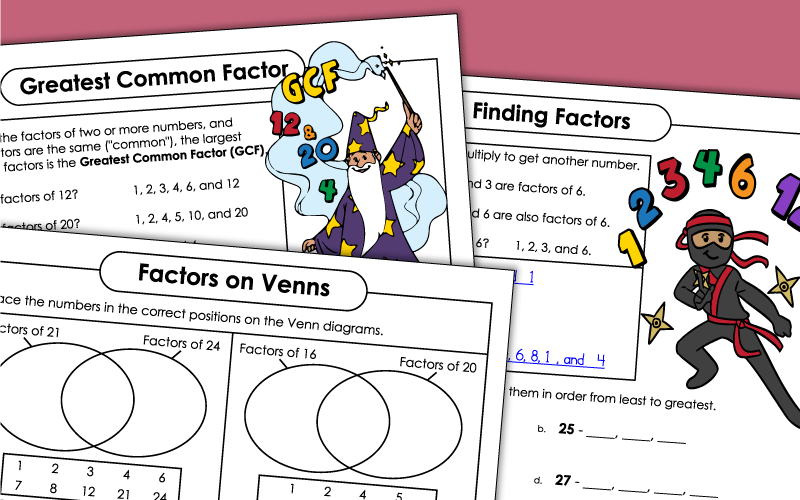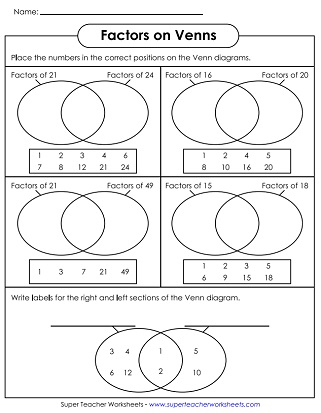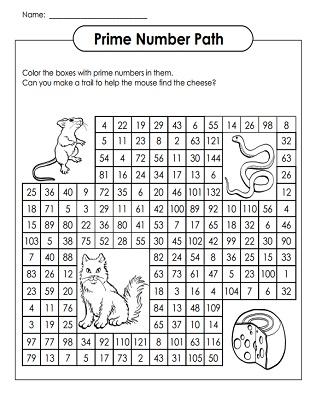# Factors

A collection of math worksheets on finding factors, greatest common factors, and factor trees, as well as prime numbers & composite numbers.## Factors

List all of the factors for the given numbers.

## Factor Trees

Find the prime factorization by completing the basic factor trees
More basic factor trees for teaching prime factorization
Complete these factor trees (different style) to reduce a number into its prime factors.

## Prime Numbers

Use the Sieve of Eratosthenes to find prime numbers. The sieve is simply a hundreds chart. Students check off multiples of 2, 3, 5, and 7. Every number that is not checked off is a prime number.

## Greatest Common Factor (GCF)

Step-by-step introduction for finding the GCF of number pairs
This file contains 15 task cards for reviewing Greatest Common Factor. Use these cards for peer review, classroom scavenger hunts, exit slips, or learning centers.

## Proper Factors andPerfect Numbers

List the proper factors of the given numbers. Proper factors are factors of a number, excluding the number itself.
List the perfect factors of the given numbers. Then determine which number is a perfect number. (A perfect number is a number whose proper factors add up to the number itself.)

Least Common Multiple (LCM) Worksheets

Worksheets in which students determine the least common multiple, or LCM, for each number set

Finding Multiples

Here you'll find resources to help students review multiples of given numbers.

Fraction Worksheets

Simplifying fractions, equivalent fractions, operations with fractions. Also includes adding, subtracting, multiplying, and dividing fractions.

Multiplication Worksheets

Practice basic multiplication skills with these printable worksheets and games

## Worksheet ImagesMy Account
Site Information